Thread: Eric Dollard View Single Postt-rexSenior Member Join Date: Aug 2011 Location: In the bushes Posts: 422
Law of Electro-Magnetic Induction, Three (1 of 2)

(1) The analysis of the Faraday Law, the Law of Electro-Magnetic Induction, continues here with the Theory and Calculation of Alternating Current Phenomena, by Charles Proteus Steinmetz, PhD, 1916. This is the fifth edition, which is partitioned into several other allied books. Two chapters are used in the analysis here, chapter three, The Law of Electro-Magnetic Induction, and chapter twenty-five, Distortion of Wave-shape and Its Cause. The chapter on reaction machines is missing from the fifth edition as it is partitioned into an allied volume. The 1900 edition is more suited for the study of Synchronous Parameter Variation in terms of a wider conception, however the 1916 edition is sufficient for the analysis here, this is all glom provides.

(2) Many of the expressions given by Steinmetz are unclear, particularly the harmonic expression of the reactance variation. The signs and symbols in the subscript have misprints, and the dimensions often do not line up. It must also be remembered that Steinmetz was forced to alter his mathematical expressions by the P.E.E.E. He literally came under attack by the pendants particularly for his development of the Law of Hysteresis. Hence Steinmetz changed his expressions because of PEEE adopted international standards, to quote;

Thus for the engineer familiar with one representation only, but less familiar with the other, the most convenient way when meeting with a treatise is in, to him, unfamiliar representation is to consider all the diagrams clockwise and all the signs of j reversed.

In conformity with the recommendation of the Turin Congress  however ill conceived this may appear to many engineers  in the following the Crank Diagram will be used, and where ever conditions require the Time Diagram, the latter be translated into the Time Diagram.

In basic terms if it seems daylight savings time is a bad idea, now it is a standard that all clocks must turn backwards in their rotation.

(3)Steinmetz does not use rational units in the development of his expressions. Heaviside warns that this is fatal to a solid theoretical understanding. The result is a quagmire of four-pi, one over c square, one over ten and all multiplied by ten to the eighth power. Steinmetz compounds the confusion by using cyclic frequencies in cycles per second rather than angular frequencies in radians per second. This results in the continual appearance of two-pi in all his expressions. The numeric pi is seen to appear and vanish so many times in Steinmetz equations and formulae that its meaning has become lost.

Steinmetz works backward from the practitioner's law for the output of a D.C. dynamo,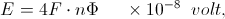Where E is the developed E.M.F., F is the frequency in cycles per second, n is the number of turns, and phi is the quantity of magnetism. The numeric 4 is the number of inductions per cycle, one for each angular quadrant. Steinmetz considers this as an average E.M.F. where in actuality the average value of a sine wave is zero. His average value is the rectified value, that of a half wave. This in order to express the E.M.F. in maximum or peak values, the factor pi over two must be affixed to the average E.M.F. This creates another pi in all the expressions, fading in and out like a ghost thruout.

In the development of his expressions, by working backwards, it appears to be a force-fit in order to arrive at an already predetermined result, a form of contrivance to quickly reach a practical result. This obviates any understanding of the theoretical conditions involved. Steinmetz created his system of mathematics for the practicing engineer, not the theoretician. This riles the pendant, nemesis ensues.

(4) As is well known Steinmetz introduced many mathematical concepts to greatly simplify the understanding and utilization of alternating current electricity. But General Electric did not want him to put too much know how in his books. Other than Steinmetz, it was only Nikola Tesla of Westinghouse Electric that could make working A.C. equipment. But Tesla did not write books, his failing, out loss.

Thruout his writings Steinmetz utilizes a dimensionless time operator which greatly simplifies the expression of alternating current phenomena. An angular rate is substituted for a time rate. It is an instantaneous position variable along the A.C. cycle, the dimension of time is canceled by the angular frequency in radians per time, given is,

t = Time Variable, second

T = Time Period, second

F = Frequency, cycle per second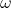= Time Rate, per second

Hence the following expressions,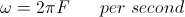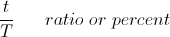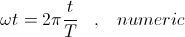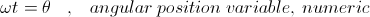Where the factor 2pi can be called an angular tensor.

The differential expressions for alternating current phenomena become a dimensionless operator, not a time rate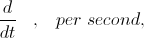is now,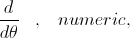Now it is a variation in angular position, radians are dimensionless.
__________________
SUPPORT ERIC DOLLARD'S WORK AT EPD LABORATORIES, INC.

Purchase Eric Dollard's Books & Videos - 70% of the sale goes to Eric and EPD Laboratories: Eric Dollard Books & Videos
Donate by Paypal: Donate to EPD Laboratories